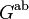# Second cohomology group for trivial group action of S4 on Z2

This article gives information about the second cohomology group for trivial group action (i.e., the second cohomology group with trivial action) of the group symmetric group:S4 on cyclic group:Z2. The elements of this classify the group extensions with cyclic group:Z2 in the center and symmetric group:S4 the corresponding quotient group. Specifically, these are precisely the central extensions with the given base group and acting group.
The value of this cohomology group is Klein four-group.
Get more specific information about symmetric group:S4 |Get more specific information about cyclic group:Z2|View other constructions whose value is Klein four-group

## Description of the group$\! H^2(G;A)$

where$G$ is symmetric group:S4 (the symmetric group on a set of size four) and$A$ is cyclic group:Z2.$G$ itself has order 24 and$A$ has order 2.

The cohomology group$H^2(G;A)$ is isomorphic to the Klein four-group.

## Computation of the group

See group cohomology of symmetric group:S4#Cohomology groups for trivial group action. It is clear that we have:$H^2(G;A) \cong A/2A \oplus \operatorname{Ann}_A(2)$

In the case that$A$ is cyclic of order two, both the groups$A/2A$ and$\operatorname{Ann}_A(2)$ are cyclic of order two, so their direct sum is the Klein four-group.

## Elements

Note that in all cases, the base of the group extension is characteristic in the whole group because it is precisely the center of the whole group. This is because the quotient group is a centerless group and hence cannot partake of any of the center.

Cohomology class type Number of cohomology classes Corresponding group extension Second part of GAP ID (order is 48) Stem extension? Base characteristic in whole group? Derived length of extension group Subgroup information
trivial 1 direct product of S4 and Z2 48 No Yes 3 center of direct product of S4 and Z2
nontrivial and symmetric 1 special linear group:SL(2,Z4) 30 No Yes 3 center of special linear group:SL(2,Z4)
non-symmetric 1 binary octahedral group, also denoted as$2 \cdot S_4^-$, the double cover of symmetric group of type "-" 28 Yes Yes 4 center of binary octahedral group
non-symmetric 1 general linear group:GL(2,3), also denoted as$2 \cdot S_4^+$, the double cover of symmetric group of type "+" 29 Yes Yes 4 center of general linear group:GL(2,3)
Total (--) 4 -- -- -- -- -- --

## Direct sum decomposition

For background information, see formula for second cohomology group for trivial group action in terms of second homology group and abelianization

### General background

We know from the general theory that there is a natural short exact sequence:$0 \to \operatorname{Ext}^1_{\mathbb{Z}}(G^{\operatorname{ab}},A) \to H^2(G;A) \to \operatorname{Hom}(H_2(G;\mathbb{Z}),A) \to 0$

where$G^{\operatorname{ab}}$ is the abelianization of$G$ (i.e., the quotient of$G$ by its derived subgroup) and$H_2(G;\mathbb{Z})$ is the Schur multiplier of$G$.

The image of$\operatorname{Ext}^1$ in$H^2$ comprises precisely the extensions where the derived subgroup of$G$ (in this case A4 in S4) splits as a direct product and the quotient group sits in an abelian fashion on top of the center. We also know, again from the general theory, that the short exact sequence above splits. However, there is no generic natural choice of the splitting. There need not in general be a natural or even an automorphism-invariant choice of splitting.

### In this case

In this case, the image of$\operatorname{Ext}^1(G^{\operatorname{ab}},A)$ is cyclic group:Z2 (because$G^{\operatorname{ab}}$ itself is isomorphic to cyclic group:Z2) and comprises the extensions direct product of S4 and Z2 and special linear group:SL(2,Z4).

The quotient group, namely$\operatorname{Hom}(H_2(G;\mathbb{Z}),A)$, is also isomorphic to cyclic group:Z2, because$H_2(G;\mathbb{Z})$ is isomorphic to cyclic group:Z2.

The non-identity coset of the image of$\operatorname{Ext}^1$ in$H^2$ comprises the two stem extensions binary octahedral group and general linear group:GL(2,3).

There are two different choices of complement to this that we can use to split the sequence. The two ways of splitting are both automorphism-invariant and are depicted below. The rows represent cosets of the image of$\operatorname{Ext}^1$ and the columns represent cosets of the chosen complement. The first row and first column respectively represent the subgroups themselves.

First way of splitting:

 direct product of S4 and Z2 special linear group:SL(2,Z4) binary octahedral group general linear group:GL(2,3)

Second way of splitting:

 direct product of S4 and Z2 special linear group:SL(2,Z4) general linear group:GL(2,3) binary octahedral group

### More on the mapping from$\operatorname{Ext}^1$

Below is an explicit description of the mapping from$\operatorname{Ext}^1(G^{\operatorname{ab}},A)$ to$H^2(G;A)$, in terms of the original extension for cyclic group:Z2 (the abelianization of symmetric group:S4) on top of cyclic group:Z2 and what the new extension looks like for symmetric group:S4 on top of cyclic group:Z2.

Cohomology class type Number of cohomology classes Element of$\operatorname{Ext}^1$ as an extension Image in$H^2(G;A)$
trivial 1 Klein four-group direct product of S4 and Z2
nontrivial 1 cyclic group:Z4 special linear group:SL(2,Z4)